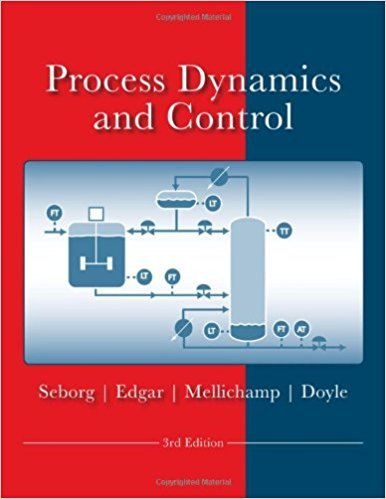×
Get Full Access to Process Dynamics And Control - 3 Edition - Chapter 4 - Problem 4.7
Get Full Access to Process Dynamics And Control - 3 Edition - Chapter 4 - Problem 4.7

×

# A single equilibrium stage in a distillation column 1sISBN: 9780470128671 148

## Solution for problem 4.7 Chapter 4

Process Dynamics and Control | 3rd Edition

• Textbook Solutions
• 2901 Step-by-step solutions solved by professors and subject experts
• Get 24/7 help from StudySoup virtual teaching assistantsProcess Dynamics and Control | 3rd Edition

4 5 1 289 Reviews
25
4
Problem 4.7

A single equilibrium stage in a distillation column 1s shown in Fig. E4.7. The model that describes this stage is dH dt = Lo + Vz - (L1 + V1) dHx1 ----;j{ = LoXo + VzYz - (L1x1 + VlYl) Yl = ao + a1x1 + azxt + ay~:f (a) Assuming that the molar holdup H in the stage is constant and that equimolal overflow holds, for a mole of vapor that condenses, one mole of liquid is vaporized, simplify the model as much as possible. (b) Linearize the resulting model and introduce deviation variables. (c) For constant liquid and vapor flow rates, derive the four transfer functions relating outputs x1 and Yl to inputs xo and yz. Put in standard form.

Step-by-Step Solution:

Problem 4.7A single equilibrium stage in a distillation column 1s shown in Fig. E4.7. The model thatdescribes this stage is dH = L0 V -2L +1 ) 1 dt dHx 1 = L00 V Y2-2L x1 1 y 1 1 dt y1= a0 a x1 0 x2 1+ a3 1 (a) Assuming that the molar holdup H in the stage is constant and that equimolal overflowholds, for a mole of vapor that condenses, one mole of liquid is vaporized, simplify themodel as much as possible. (b) Linearize the resulting model and introduce deviationvariables. (c) For constant liquid and vapor flow rates, derive the four transfer functions relatingoutputs x and y to inputs x and y . Place it in standard form. 1 1 0v 2 Step-by-step solution Step 1 of 9 ^(a)Consider the molar holdup H in the equilibrium stage is constant.Refer to Figure E4.7 in the textbook, assuming equimolal overflow. L = L = L 0 1 V1 V 2VConsider the equilibrium stage model dH . dt dH = L + V - (L + V ) dt 0 2 1 1Substitute L for L , L f0 L , V for1 and V for1 . 2 dH dt = L + V - (L + V) = 0

Step 2 of 9

Step 3 of 9

##### ISBN: 9780470128671

Unlock Textbook Solution